So I wanted to plot some data and decided to create a simple line graph using `UIBezierPath`. It worked but I wasn’t happy with it. Then I decided to use the quadratic bezier curve method that requires two end points and one control point. Still wasn’t happy with result. Then came the turn of the Cubic bezier curve, that requires two control points and two end points. I knew I needed to use this to create a curve that went through the prescribed points and also creates the smooth and perfect curve that I was looking for. However, it isn’t as straight forward as it first seems.

The bezier cubic curve method signature is this:

It requires the next end point, and then two control points. I have the end points (calculated from the data set) but not the control points. This is where my research started. I wanted to figure out how to calculate the control points of a cubic bezier and de Casteljau curve.

Before I get into the algorithm, maths and the code, I would like to say that this article will be far more theoretical and mathematical than any other article that I have posted on this website. It will contain the coding bit as well, but that will be pretty straight forward once you understand the actual logic behind calculating control points. You will need some understanding of Calculus and Linear Algebra.

Now let’s focus on how a bezier curve is actually formed. A quadratic bezier curve has two end points and one control point. The control point can be pictured as a magnet that pushes the main line towards itself giving us the “curve” that we want.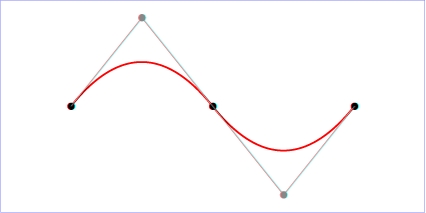As you can see we have the gray colored point that is basically acting as the control point. To go further in detail, the control point is connected to the two end points. The two lines projecting from the control point are tangent to the curve. Moving the control point will change the gradient of the two lines in turn changing the curve.

But you may ask, how does it actually work? Like it’s not actually a magnet right? Obviously, it’s not a magnet - basically we have simple coordinate geometry going on here. Let’s find out:

The two lines that are projecting from the control point and in tangent to the two end points. Now let’s take a parameter say `t` which goes from 0 to 1. Also, we will picture that there is a point on each of these gray lines. We will call them `p01` and `p12`. The `p01` is moving from endpoint `p0` to control point `p1` as `t` goes from 0 to 1. And similarly, we have a point `p12` that moves from control point `p1` to end point `p2` and `t` goes from 0 to 1.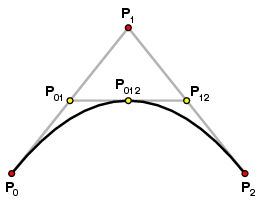Imagine there is a line drawn between the point `p01` and `p12` and there is another point on this line `p012`. This point moves from `p01` to `p12` as `t` goes from 0 to 1. So now when you start the `t` parameter, you will see that are curve is drawn with respect to the position of the control point.

This is just the basic concept of how bezier curves are formed using one control point. The problem with the quadratic control point is that it only gives us one control point and it doesn’t provide us with the flexibility that we are looking for to create a smooth curve. This same understanding can be applied to cubic bezier curve as well that contains two control points.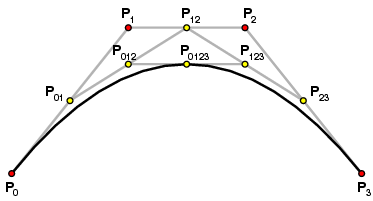To better understand how the bezier curve is drawn, take a look at this extremely well made video/animation:

### The Equation:

Now that we have the basic understanding of how bezier curves work, and can picture how the curve is drawn, now it’s time to represent this entire thing with a mathematical equation and here it is:(Eq 1)

This is the parameterized equation of a cubic bezier curve, where `t` which is the parameter can go from 0 to 1. In this equation, the point `p0` and `p3` are the end point and the point `p1` and `p2` are the control points. Also note that when `t` = 0, the output of the equation is `p0` and when `t` = 1, the output is `p3`. Now as you move the `t` from 0 to 1 (say in 0.1 increments), the control point contains more weightage in the output. Till a certain `t` one control point has more weightage and effects the curve more, and for some other range of values for `t`, the next control point has more weightage and effects the curve.

For example, when `t` = 0.3: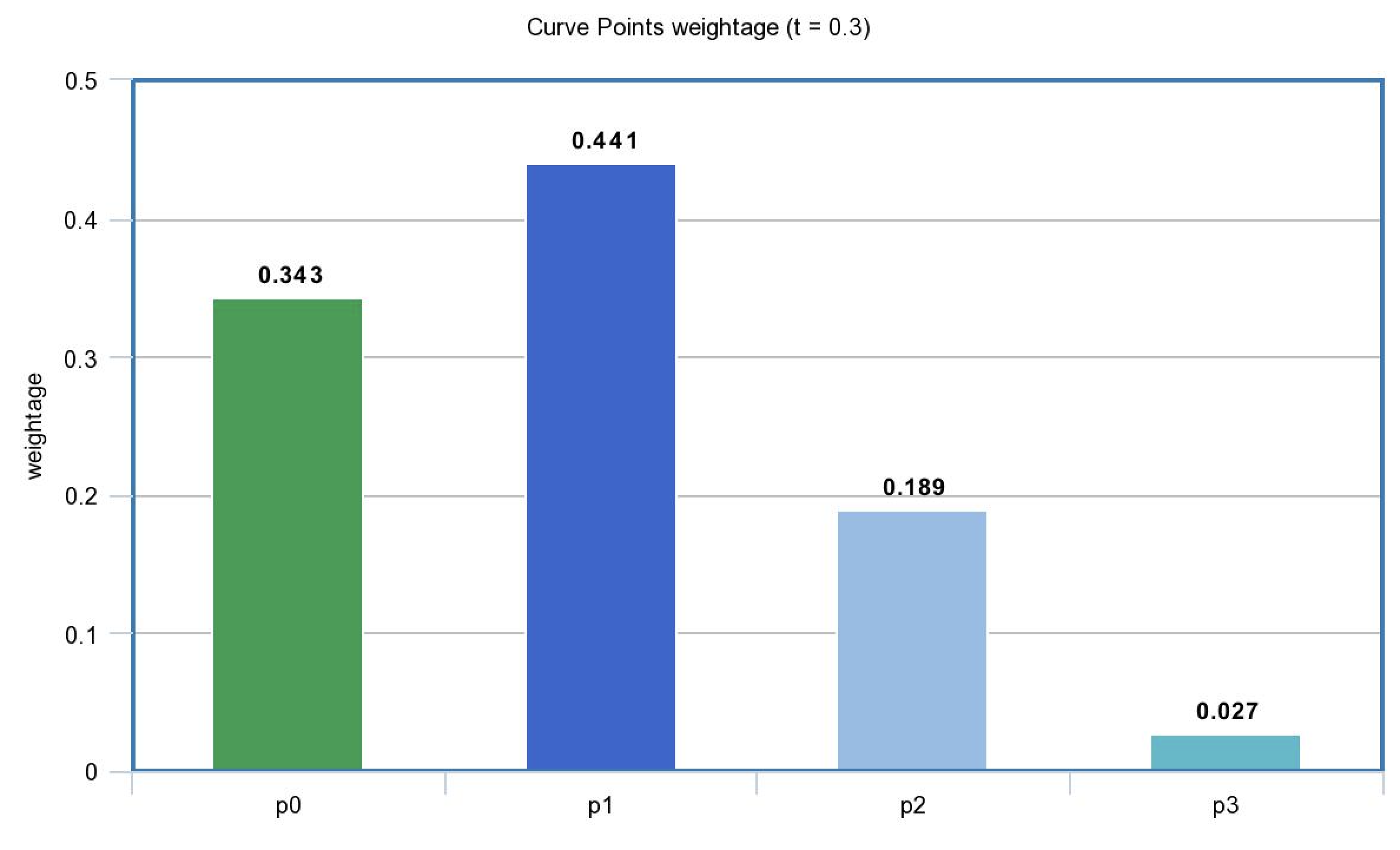when `t` = 0.7, the second control point and the second end point has the most weightage in the curve: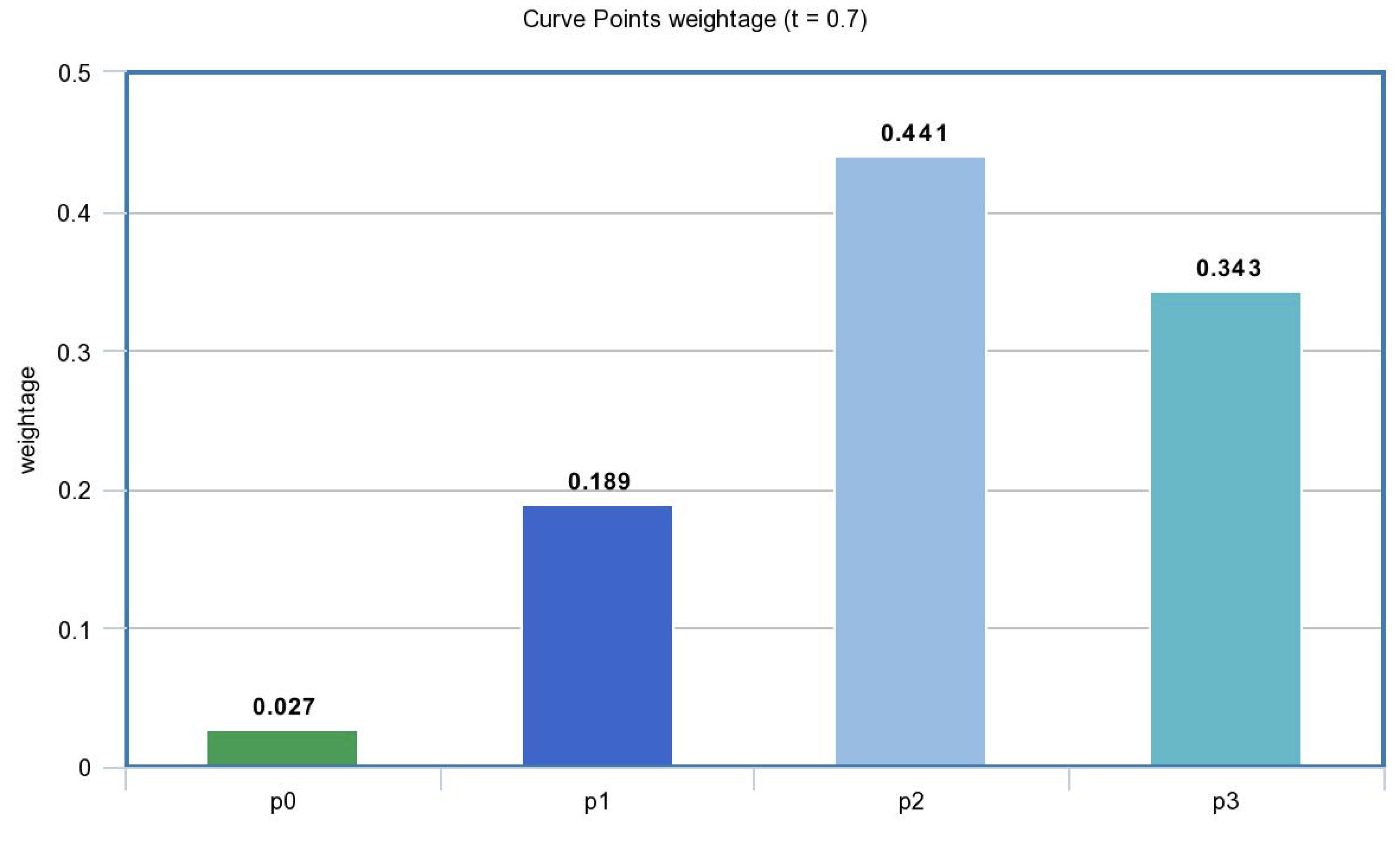Now let’s take a closer look at how to create smooth curves. The first thing we need to observe is that while this above equation is a 3rd degree polynomial, we can use higher degree polynomials as well which of course means more control points and much precise curve control.

`Number of control points = degree of polynomial - 1 `

However, higher polynomial degree means more complex computations. Therefore, we will stick with 3 degree polynomial or cubic curve. In order to get a long curve, we will stitch cubic curves together.

### Conditions:

Since multiple curves will be stitched together, they need to follow a certain protocol:

1. Each curve will have two end points (`p0` & `p3`) and two control points (`p1` & `p2`).
2. The last endpoint of one curve will be first endpoint of the next curve. (shared point)
3. To get a smooth curve we need to make sure the slope of the line connecting `p2` and `p3` of the curve is same as the slope of line connecting `P3` and `Q1` of the next curve.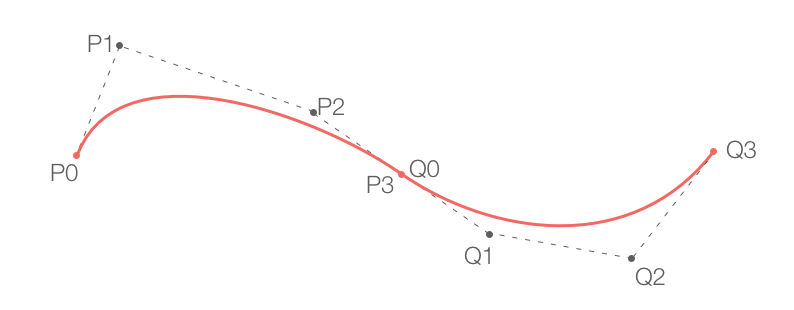Let’s talk about the derivatives now. The first derivative of any polynomial curve is it’s slope or the gradient at a particular point. The second derivative of the equation tells us about the maxima/minima and continuity behavior. We will use these derivatives to derive equations for our curve. Let’s take a closer look at the third condition of the protocol.

The slope of the line connected `p2` and `p3` - which is the second control point and last end point of curve should be same as the slope of line connecting the control point `Q1` and end point `P3`. Let’s first write the derivative of the cubic bezier equation: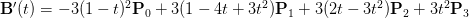(Eq 2)

Now before we go any further let’s talk about the segments and points. If you have `n` data points, and you wish to create a curve that pass through all the data points, then you shall have `n - 1` segments or curves. In the above picture you can see that there are three data points, `p0`, `p3` and `Q3` and there are 2 curves or segments (from `p0` to `p3` and from `p3` to `Q3`).

The two curves have a shared point (`p3`) and we need to make sure that the slope for both curves at this point is same. Note that `p3` is the last endpoint for the first segment and the first end point of the next segment.

As I showed earlier in the article, if we put `t = 0` in the cubic bezier equation we get the first endpoint as the output and `t = 1` yeilds the last endpoint. Similarly, in the Eq 2 (derivative equation of the cubic bezier curve) - we will put `t = 1` for the `i - 1`th segment and `t = 0` for the `i`th segment, where (`i > 0`).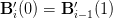(Eq 3)

Once you have substituted the correct `t` values in Eq 2 and evaluated Eq 3 you will get this equation:(Eq 4)

Do note here the second condition of our protocol, that states that the last endpoint of one curve will be first endpoint of the next curve. So point `p0` of `i` segment and point `p3` of `i - 1` segment are shared or same. We will call them as `k`. `k` from now on will represent an endpoint which is shared between two segments.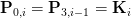Using the above identity in Equation 4 gives us the following important equation: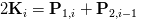(Eq 5)

We will now move to the second derivative. The second derivative tells us about the continuity of the curve. The second derivative of the bezier curve is: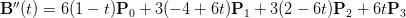(Eq 6)

The second derivative tells us if there is any discontinuity at a particular point in our graph. Since the third condition in our protocol mentions that the curve is continuous so the second derivative shall also be same at the shared point. So go ahead and substitue `t = 0` and `t = 1` in Eq 6. Make sure that you follow the same concept which was followed earlier:

Substitute `t = 0` for the `i th` segment and `t = 1` for `i - 1 th` segment in the Eq 6 and make them equal to each other. This is what you will get after simplification: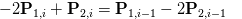(Eq 7)

This is our first of three equations which we will use to do most of our evaluation. Also keep in mind that the above equation is in terms of `P2` and `P1` - the two control points.

Now we will take a look at the extremities - where the curve starts and ends. These two points have a discontinuity or the second derivative of the curve at these points is `0`. Let’s begin by taking the very first segment of the curve. Since the extreme point is when `t = 0`, so we will substitute `t = 0` in Eq 6.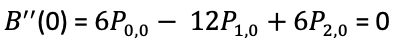In the above equation you can see we have `P0` which is an end point and not the control point, so we will substitute it with `K0` and this is the equation we will get now: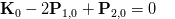(Eq 8)

Let’s do the same with `t = 1` now for the last segment which will be `n - 1 th` segment, again make sure to substitute `Kn` in place of `P3` which is an extreme end point of the last segment.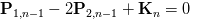(Eq 9)

We are almost there. Here is the situation right now: Using our understanding of basic calculus and a bit of algebra, we have been able to get three equations in terms of the unknowns which are the control points `P1` and `P2`. We will first try to solve for one control point and then once `P1` is found, we can find `P2`. So in Eq 7, Eq 8 & Eq 9 we have `P1` and `P2`. We will now get all these three equations in terms of `P1` and the `K` - (which is nothing but the known end points for relative segments).

In order to get this sort of an equation, we will substitute `P2` with Eq 5 and we will get the following 3 equations: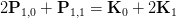(Eq 10.1)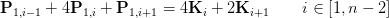(Eq 10.2)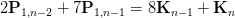(Eq 10.3)

### The Equations:

The Eq 10.1, 10.2 & 10.3 are the three mains equations that we be using. The equations are in the form of `P1` which is unknown and `K` which is just the end point. Now keep this in mind that the total number of equations will obviously depend on the number of data points. The number of equations will be same as the number of segments or total data points - 1.

Equation 10.1 is the equation to find the `P1` of the first segment. Similarly, Equation 10.3 is to find the `P1` for the last segment (n - 1). The equation 10.2 is the generic equation, and the quantity of this equation will depend upon the data set. Let’s take a closer look at Eq 10.2.

### Equation 10.2 In Depth:

The Equation 10.2 is the most important one when it comes to understanding the whole applied mathematics point of view. We have a system of equations and we will be using a bit of linear algebra to solve for the unknowns.

The `i` subscript in this equation refers to the index of the data point. For example, `i = 0` means, the first element in data array and `i = 1` means the second element of the data array. If `i = 0`, we will use the first equation (10.1), when the `i = n - 2`, this means we are dealing with second last data point in our array and so we will use the equation 10.3. For `i = 1` all the way till `i < n - 2`, we will use equation 10.2. Confused? Let’s clear it up:

Imagine we have an array p, that contains 7 data points:

Fetching first data point is pretty trivial = `p -> (6,5)`. Since in our above equations, we require data points for the right hand side only where `Ki` is the data point from the array. When we are dealing with first equation (10.1), we need the first and second element of the data set; `i = 0 & i = 1`.

Then for the last segment we need the second last element from the data point, the `i` value to fetch this will be `i = n - 2` or `i = 7 - 2 = 5`.

Now, we will use the second equation and our `i` value will go from `i = 1` to `i = 4`, which returns us the second element till the fifth element:

The above example should help us when we are coding this whole thing up.

### The Matrix

Now is the time to create a matrix where we will use the above equations and create a tri-diagonal matrix. For this example we will assume that we have 7 points, so the total segments will be (7 - 1) = 6. Which means our matrix A will be 6 x 6. Let’s start with Eq 10.1 and Eq 10.3 first: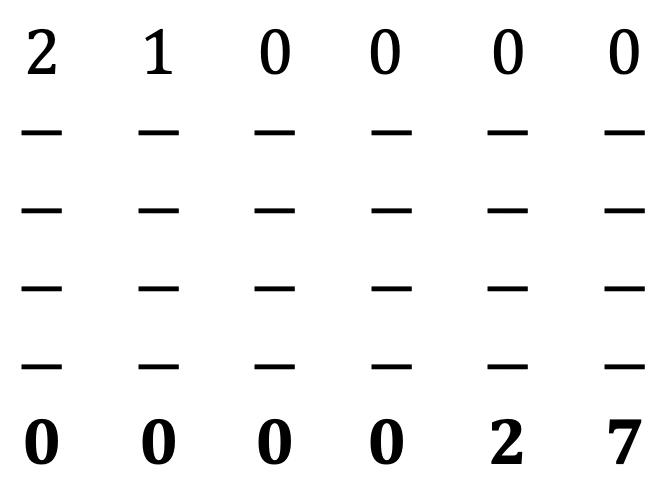(M1.0)

We are only concerned with the left hand side of the equations for now.

Now let’s begin adding the remaining elements. The remaining elements will be filled by using the Eq 10.2. The picture below shows the full tri-diagonal matrix: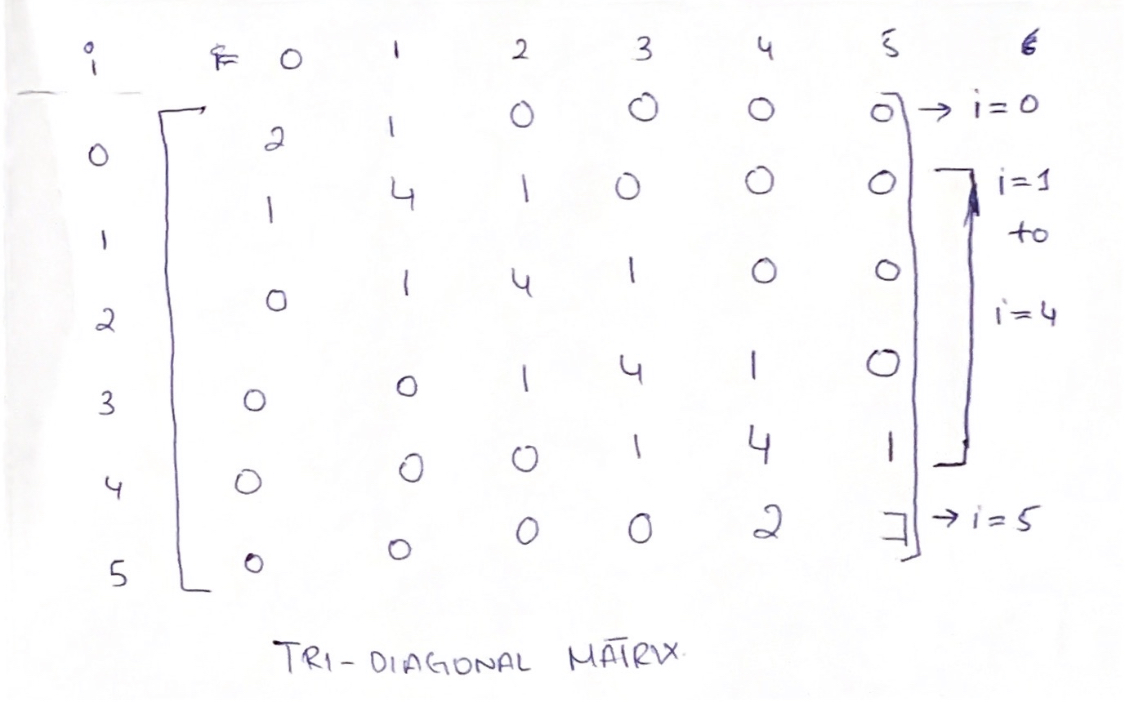The tridiagonal matrix essentially contains three diagonals, one main diagonal and then one on top and one on the bottom of the main diagonal. Such matrices are quite easy to compute and takes less computational resources. So now we have the left hand side of the equation. The right hand side is just the data points. Now we will use the Thomas Algorithm to calculate for P1. `A * P1 = B`

So this is how our system will look like: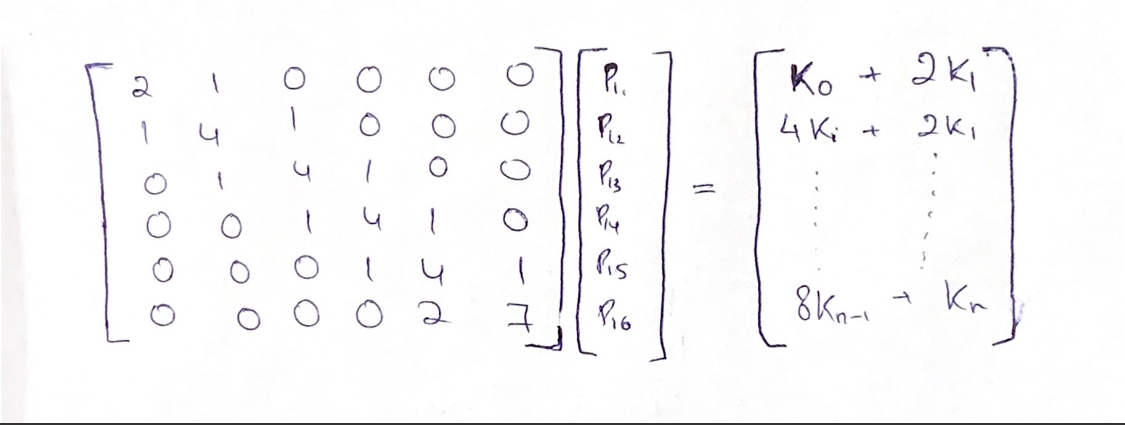### Thomas Algorithm:

Before we get into the coding bit, let’s use the Thomas Algorithm to calculate the unknown `P1's`. Thomas Algorithm work by working with the three diagonals only and doing simple row operations on them.

The first thing we will do is to make the first element of the main diagonal `d` = 1.To do this, we divide first row by `d1` and we get:

`a1' -> a1 / d1`

`B1' -> b1 / d1`

Next, we will use the first row and use it as a pivot to eliminate the element b2: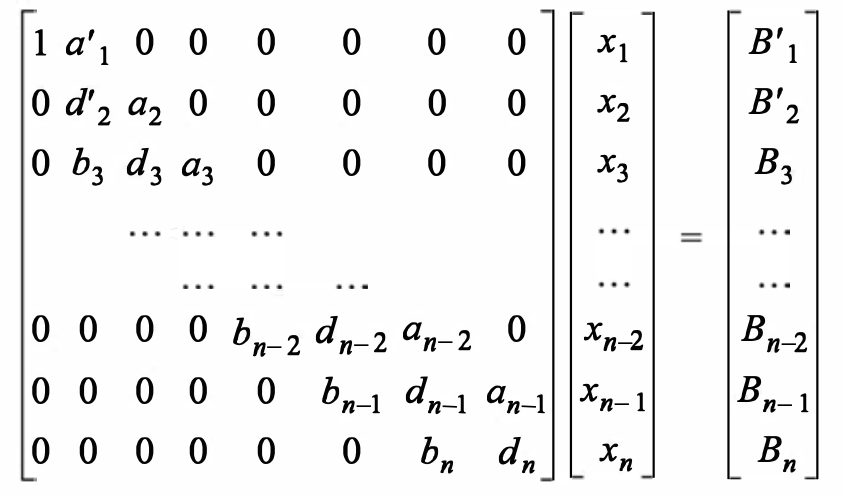We do this by multiplying first row with `b2` and then is subtracted with the second row, we get:

`d2' -> d2 - b2a1'`

`B2' -> B2 - B1b2`

We will do the same operations for all the remaining rows, eliminating essentially the bottom diagonal. So basically we will normalize the second row by dividing the 2nd row with `d2'` which makes the element `d2' = 1`. Then we will use this element as the pivot and multiply the entire row with `b3` and then is subtracted with the third row, eliminating the `b3` element in the below diagonal.

In the end we will get such a matrix: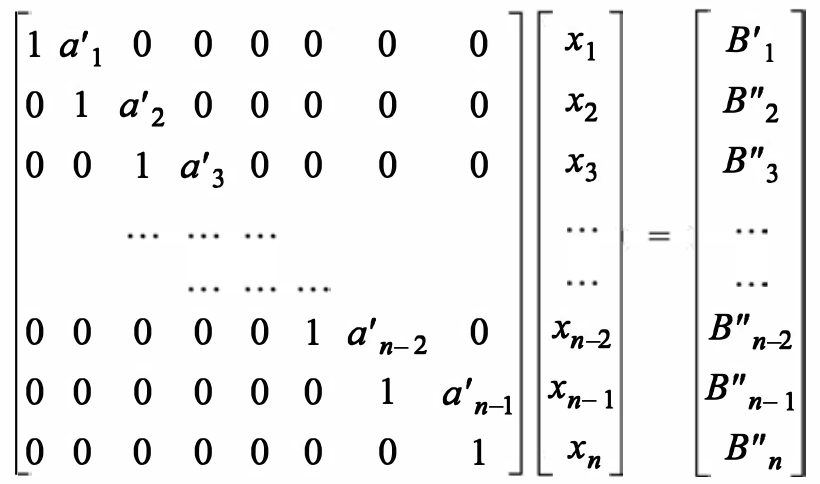The last row’s last column is 1, which allows for simple back substitution and finding all the `p1's`.

Let’s see how we can make all these operations generic, so that we can use a simple for loop and have all these operations take place.

Take three arrays for three diagonals

When `i = 1`, we will use:

`a1'` = `a1 / d1`

`B1'` = `B1 / d1`

For `i = 2 to i = n - 1`: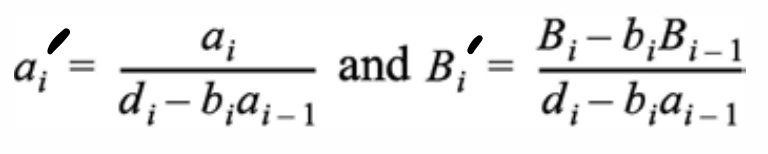and when `i = n`: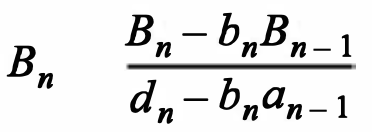At the end we will do the back substitution to get the values for unknown. We will do this step once we get to the coding portion. Now that we have our `P1` control point, we can use these points to get the other control point `P2`. To get `P2`, we will use Eq 5 and Eq 9.

If we make `P2` the subject we get the following two equations: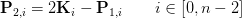(Eq 11.1)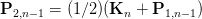(Eq 11.2)

That’s all the maths that we need to do. So now we have the system of equations for `P1`, and also the equations for `P2`. Next, we have discussed the thomas algorithm which will help us solve for the system of equations. Now it’s all about the code. The code is pretty straight forward. Let’s code it all out 🚀

### Let's Code:

The above block of code basically states that there is a new class called `BezierConfiguration` that contains a `configureControlPoints(data: [CGPoint])` method that takes in an array of `CGPoint`and returns a custom type of `BezierSegmentControlPoints` that houses the first and second control point per segment.

In side the function, we are first grabbing the total number of segments. If there is just 1 segment or 2 data points, then there will be a straight line and here I am sending the end points as the control points, so in essence there should be no curve on the line.

If segments are more than 1, then we will do the following:

In this block of code, we are first creating three arrays, one for the main diagonal `d`, then one for the diagonal above the main one, `ad` and one for below, `bd`. Then we will create a `rhsArray` that will house all the coefficients for the right hand side of our system of equations. The `d`, `ad` and `bd` will house all the coefficients for the left hand side of the system of equations. Again we are using `i` to keep track of the index for our original points in the data set. So now we run the for loop that will go from `0` to `n - 2` or `number of segments - 1`.

We need to be careful here not to run a loop where the `i` is not in the range of the array, so make sure you understand why we are running the for loop till a certain value.

At the start of each iteration, we are calculating the two endpoints for each segment `p0` and `p3`. Then if it is the first point `i = 0`, then we use the `Eq 10.1`. we append the respective coefficients to `bd`, `d` and `ad` and the `rhsXValue` and `rhsYValue`.

If the index value/ `i` is equal to `segments - 1`, or the second last point, then we will append the respective coefficients to the diagonal arrays and also create the `rhsXValue` and `rhsYValue` for that equation. Lastly, we have all the segments between the first and last segment. Again we do the same thing and append the coefficients to the diagonals arrays and create the respective `rhsXValue` and `rhsYValue` which then we append to the `rhsArray`.

Thus far, we have created a system of equations. We have the right hand side and left hand side of the equations. Now we will implement the Thomas algorithm. So let’s create a new function and send the relevant data to the function:

In this function, we are getting all the diagonal matrices, the number of segments and the right hand side array and of course our data values. Now we will implement the thomas algorithm. It’s pretty simple and we will just be implementing the equations that we have already discussed earier:

The first thing we do inside this function is create a `solutionSet1` array and initialize the array with specific number of elements. Next we will solve the first equation, and for that we simply implement the equation already mentioned earlier. The `B` array that we took in our Thomas Algorithm section will now be replaced with the `rhsArray` and since we have a point here, so make sure you do the operation to both the `x` and `y` coordinates.

Next we will do this for the middle segments or equations. One thing to note here is the fact that we am using the if statement to check if the `segments` are more than 2. If there are 2 segments only, then we only want the first segment and the last segment code to run.

Again we are simplying creating a for loop and iterating through all the remaining `rhsArray` and diagonals coefficients. Lastly, we will implement the last equation:

Now, that we have implemented the algorithm, it’s time to do the back substitution. The beauty of this whole Thomas algorithm is that it provides us with the value of the last unknown variable `P1(6)` and then we will substitute this to the equation above it and keep doing it to find all the unknown. So here we will start with a for loop that is reversed, so it starts with last index value and goes all the way back.

The reason why we initialized the solutionSet1 to `nil` but with a specific count was so that we can add the last `rhsArray` element in the last position of the solution set:

So we start a for loop again but reversed, and then find the remaining control points (unknown points) and append it to the `solutionSet1` array. Now we have all the first control points. Next we will use the equation 11.1 and 11.2 to find `p2`.

Now we have two arrays one with all the first control points and the other with second control points. Now we will create any array of `BezierSegmentControlPoints` where there will `P1` and `P2` for each segement.

### UI and Implementation

In the view controller, create an array of data points and instance of `UIBezierPath`:

In the `ViewDidLoad`:

So now we have all the control points. Now after you have grabbed all the control points, start a for loop and create a bezier path:

If `i == 0`, then we will move the `bezierPath` to that point or in other words start the bezier path. Then for all the remaining points we will grab the segement from the control points array and hen use the `addCurve` method on `bezierPath` so provide it with the next point and the control points.

Lastly, we will create the `CAShapeLayer` and give it some color and animation:

And that’s it! We are done 🎉🚀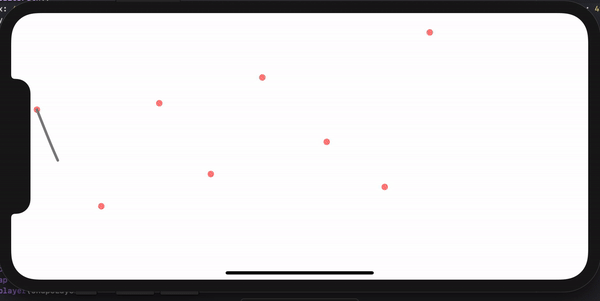⭐️ The full project is available on my Github